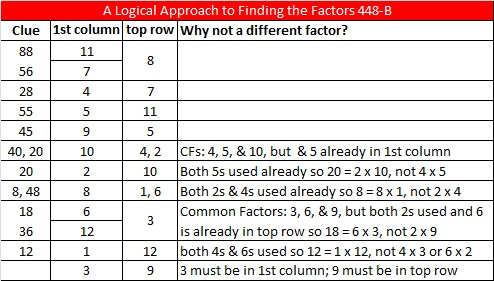# 672 Is it too soon to pick out a tree?

672 can make MANY factor trees.  Here I’ve pictured only a few of the possibilities, one for each of its factor pairs (excluding 1 x 672).

Is it too soon to pick out a tree?Every one of those trees has the prime factors of 672: 2, 2, 2, 2, 2, 3, and 7, but finding them on each tree might be a challenge because I didn’t distinguish the prime factors from the other factors. Some of those prime factors might seem like they are lost in a pile of leaves. Can you find them on each tree?

672 is the 9th number to have 24 factors. Here is a number line highlighting all nine of those numbers and the distances between them.Notice the difference between 672 and the previous number with 24 factors is 12, a record low.

You might get the impression looking at the number line that numbers having 24 factors might be much more common from now on. That may be true, nevertheless, the next number after 672 to have exactly 24 factors is 756 which is 84 numbers away and well past 720 the smallest number to have 30 factors.

Indeed infinitely many numbers have 24 factors, but probably 672 is the last one that will get much attention.

The numbers in the factor pair 24 and 28 are each exactly two numbers away from 26, their average. That means we are just 2² numbers away from 26².

In other words, 672 equals 26² – 2² which can be factored into (26 + 2)(26 – 2) so (26 + 2)(26 – 2) = 28 x 24 = 672.

• 672 is a composite number.
• Prime factorization: 672 = 2 x 2 x 2 x 2 x 2 x 3 x 7, which can be written 672 = (2^5) x 3 x 7
• The exponents in the prime factorization are 5, 1, and 1. Adding one to each and multiplying we get (5 + 1)(1 + 1)(1 + 1) = 6 x 2 x 2 = 24. Therefore 672 has exactly 24 factors.
• Factors of 672: 1, 2, 3, 4, 6, 7, 8, 12, 14, 16, 21, 24, 28, 32, 42, 48, 56, 84, 96, 112, 168, 224, 336, 672
• Factor pairs: 672 = 1 x 672, 2 x 336, 3 x 224, 4 x 168, 6 x 112, 7 x 96, 8 x 84, 12 x 56, 14 x 48, 16 x 42, 21 x 32, or 24 x 28
• Taking the factor pair with the largest square number factor, we get √672 = (√16)(√42) = 4√42 ≈ 25.92296.# 650 is the sum of all the clues in this Level 1 puzzle

1² + 2² + 3² + 4² + 5² + 6² +7² + 8² + 9² +10² + 11² + 12²  = 650

Thus 650 is the 12th square pyramidal number and can be calculated using 12(12 +1)(2⋅12 + 1)/6.

If you add up all the clues in today’s Find the Factors puzzle, you will get the number 650. However, if you print the puzzle from the excel file, one of the clues is missing because it isn’t needed to find the solution.

650 is the hypotenuse of seven Pythagorean triples!

• 72-646-650
• 160-630-650
• 182-624-650
• 250-600-650
• 330-560-650
• 390-520-650
• 408-506-650

Can you find the greatest common factor of each triple? Each greatest common factor will be one of the factors of 650 listed below the puzzle.

650 is the hypotenuse of so many Pythagorean triples because it is divisible by 5, 13, 25, 65, and 325 which are also hypotenuses of triples. The smallest three numbers to be the hypotenuses of at least 7 triples are 325, 425, and 650.

Since 25 x 26 = 650, we know that (25-1)(26 + 1) = 650 – 2. Thus 24 x 27 = 648.Print the puzzles or type the solution on this excel file: 12 Factors 2015-10-19

—————————————————————————————————

• 650 is a composite number.
• Prime factorization: 650 = 2 x 5 x 5 x 13, which can be written 650 = 2 x (5^2) x 13
• The exponents in the prime factorization are 1, 2, and 1. Adding one to each and multiplying we get (1 + 1)(2 + 1)(1 + 1) = 2 x 3 x 2 = 12. Therefore 650 has exactly 12 factors.
• Factors of 650: 1, 2, 5, 10, 13, 25, 26, 50, 65, 130, 325, 650
• Factor pairs: 650 = 1 x 650, 2 x 325, 5 x 130, 10 x 65, 13 x 50, or 25 x 26
• Taking the factor pair with the largest square number factor, we get √650 = (√25)(√26) = 5√26 ≈ 25.495098.—————————————————————————————————

# 640 Fall Factor Trees and Level 5

Because it is fall, and 640 has many factors, I decided to make factor trees using fall colors. Get out your rakes!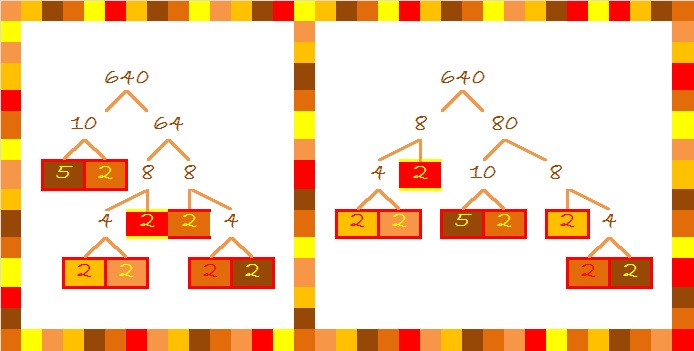There are many other possible factor trees for 640, but raking leaves can be a lot of work, so I only made two of them.

640 is the hypotenuse of the Pythagorean triple 384-512-640.

OEIS.org informs us that 640 = 16!!!!!!, but if you type 16!!!!!! into a calculator, you will get an error message as soon as you type !!.

16!!!!!! ≠ (((((16!)!)!)!)!)!

There are 6 !’s so 16!!!!!! = 16(16-6)(16-12) = 16 x 10 x 4.

Here is this week’s Level 5 puzzle: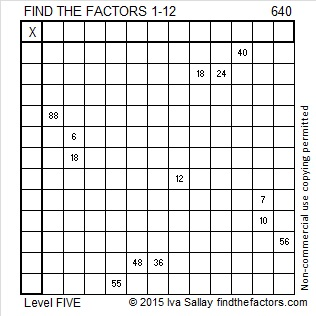Print the puzzles or type the solution on this excel file: 12 Factors 2015-10-05

—————————————————————————————————

• 640 is a composite number.
• Prime factorization: 640 = 2 x 2 x 2 x 2 x 2 x 2 x 2 x 5, which can be written 640 = (2^7) x 5
• The exponents in the prime factorization are 7 and 1. Adding one to each and multiplying we get (7 + 1)(1 + 1) = 8 x 2 = 16. Therefore 640 has exactly 16 factors.
• Factors of 640: 1, 2, 4, 5, 8, 10, 16, 20, 32, 40, 64, 80, 128, 160, 320, 640
• Factor pairs: 640 = 1 x 640, 2 x 320, 4 x 160, 5 x 128, 8 x 80, 10 x 64, 16 x 40, or 20 x 32
• Taking the factor pair with the largest square number factor, we get √640 = (√64)(√10) = 8√10 ≈ 25.298221—————————————————————————————————# 630 Factor Trees and Level 2

### Today’s Puzzle:Print the puzzles or type the solution on this excel file: 10 Factors 2015-09-28

### Factors for Today’s Puzzle:### Factor Trees for 630:

630 is the 7th number with exactly 24 factors. So far, the seven numbers counting numbers with 24 factors are 360, 420, 480, 504, 540, 600, and 630. No counting number less than 630 has more than 24 factors.

Two of those seven numbers make up the Pythagorean triple 378-504-630. Which factor of 630 is the greatest common factor of those three numbers in the triple?

Here are a few of the MANY possible factor trees for 630.### Factors of 630:

• 630 is a composite number.
• Prime factorization: 630 = 2 x 3 x 3 x 5 x 7, which can be written 630 = 2 x (3^2) x 5 x 7
• The exponents in the prime factorization are 1, 2, 1, and 1. Adding one to each and multiplying we get (1 + 1)(2 + 1)(1 + 1)(1 + 1) = 2 x 3 x 2 x 2 = 24. Therefore 630 has exactly 24 factors.
• Factors of 630: 1, 2, 3, 5, 6, 7, 9, 10, 14, 15, 18, 21, 30, 35, 42, 45, 63, 70, 90, 105, 126, 210, 315, 630
• Factor pairs: 630 = 1 x 630, 2 x 315, 3 x 210, 5 x 126, 6 x 105, 7 x 90, 9 x 70, 10 x 63, 14 x 45, 15 x 42, 18 x 35, or 21 x 30
• Taking the factor pair with the largest square number factor, we get √630 = (√9)(√70) = 3√70 ≈ 25.09980.### Sum-Difference Puzzle:

630 has twelve factor pairs. One of the factor pairs adds up to 53, and a different one subtracts to 53. If you can identify those factor pairs, then you can solve this puzzle!### More about the Number 630:

630 is the sum of the six prime numbers from 97 to 113.

630 is the 35th triangular number because (35 x 36)/2 = 630. It is also the 18th hexagonal number because 18(2 x 18 – 1) = 630.

630 is a triangular number that is a multiple of other triangular numbers in more ways than you probably want to know:

• 630 is three times the 20th triangular number, 210, because 3(20 x 21)/2 = 630.
• 630 is 6 times the 14th triangular number, 105, because 6(14 x 15)/2 = 630.
• 630 is 14 times the 9th triangular number, 45, because 14(9 x 10)/2 = 630.
• 630 is 30 times the 6th triangular number, 21, because 30(6 x 7)/2 = 630.
• 630 is 42 times the 5th triangular number, 15, because 42(5 x 6)/2 = 630.
• 630 is 63 times the 4th triangular number, 10, because 63(4 x 5)/2 = 630.
• 630 is 105 times the 3rd triangular number, 6, because 105(3 x 4)/2 = 630.
• 630 is 210 times the 2nd triangular number, 3, because 210(2 x 3)/2 = 630.
• and finally, 630 is 630 times the 1st triangular number, 1, because 630(1 x 2)/2 = 630.

# 420 Factor Trees

Did you know that the sum of all the prime numbers between 100 and 110 equals 420? Yes, 101 + 103 + 107 + 109 = 420.

Since 20 × 21 = 420, we know that 420 is the sum of the first 20 EVEN numbers. Thus,

• 2 + 4 + 6 + 8 + 10 + 12 + 14 + 16 + 18 + 20 + 22 + 24 + 26 + 28 + 30 + 32 + 34 + 36 + 38 + 40 = 420.

420 is the smallest number that can be divided evenly by all the natural numbers from 1 to 7.

420 has a LOT of factors, more than most people would think it does.  In fact, of all of the numbers from 1 to 420, there is only one number, 360, that has as many factors as 420 has.

There are 4 different prime numbers that can divide evenly into 420. Here are those factor trees:Many, but not all, of the factors of 420 are listed somewhere on those four trees.

• 420 is a composite number.
• Prime factorization: 420 = 2 x 2 x 3 x 5 x 7, which can be written 420 = (2^2) x 3 x 5 x 7
• The exponents in the prime factorization are 2, 1, 1, and 1. Adding one to each and multiplying we get (2 + 1)(1 + 1)(1 + 1)(1 + 1) = 3 x 2 x 2 x 2 = 24. Therefore 420 has exactly 24 factors.
• Factors of 420: 1, 2, 3, 4, 5, 6, 7, 10, 12, 14, 15, 20, 21, 28, 30, 35, 42, 60, 70, 84, 105, 140, 210, 420
• Factor pairs: 420 = 1 x 420, 2 x 210, 3 x 140, 4 x 105, 5 x 84, 6 x 70, 7 x 60, 10 x 42, 12 x 35, 14 x 30, 15 x 28, or 20 x 21
• Taking the factor pair with the largest square number factor, we get √420 = (√4)(√105) = 2√105 ≈ 20.4939Each factor pair, except 1 x 420, can make its own factor tree. Here are some factor trees featuring the other seven factor pairs:If I hadn’t made all the prime numbers in red, it’s possible that one or more of the prime numbers might get forgotten. That is why I prefer the cake method for finding the prime factorization of a number. All of the prime numbers are listed in numerical order on the outside of the cake.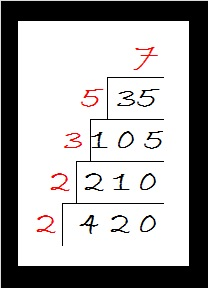# 326 Tiny Christmas Factor Tree

• 326 is a composite number.
• Prime factorization: 326 = 2 x 163
• The exponents in the prime factorization are 1 and 1. Adding one to each and multiplying we get (1 + 1)(1 + 1) = 2 x 2 = 4. Therefore 326 has exactly 4 factors.
• Factors of 326: 1, 2, 163, 326
• Factor pairs: 326 = 1 x 326 or 2 x 163
• 326 has no square factors that allow its square root to be simplified. √326 ≈ 18.055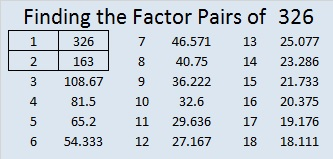Even though 326 is a three digit number, there is only one way to construct its factor tree, two if you count its mirror image. Either way is illustrated here. Below them is a Christmas factor tree puzzle that is a lot more interesting than the factor tree for 326.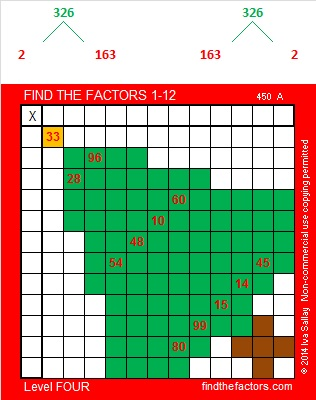Print the puzzles or type the factors on this excel file: 12 Factors 2014-12-15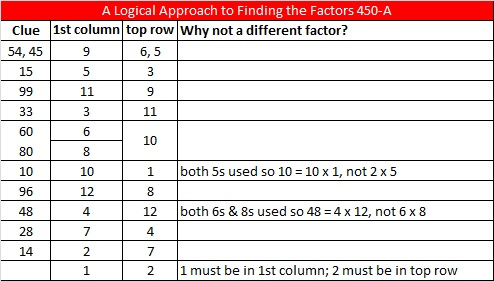# 324 Christmas Factor Trees

• 324 is a composite number.
• Prime factorization: 324 = 2 x 2 x 3 x 3 x 3 x 3, which can be written 324 = (2^2) x (3^4)
• The exponents in the prime factorization are 2 and 4. Adding one to each and multiplying we get (2 + 1)(4 + 1) = 3 x 5 = 15. Therefore 324 has exactly 15 factors.
• Factors of 324: 1, 2, 3, 4, 6, 9, 12, 18, 27, 36, 54, 81, 108, 162, 324
• Factor pairs: 324 = 1 x 324, 2 x 162, 3 x 108, 4 x 81, 6 x 54, 9 x 36, 12 x 27, or 18 x 18
• 324 is a perfect square. √324 = 18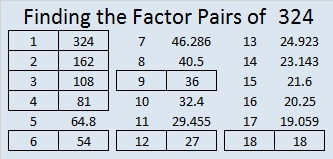Included in these 324 factor trees are smaller factor trees for the numbers in brown: 4, 6, 9, 12, 18, 27, 36, 54, 81, 108, and 162. Prime factors of 324 are in red.This Level 2 puzzle looks like part of a Christmas tree branch. It’s not too difficult to solve. Give it a try!Print the puzzles or type the factors on this excel file: 12 Factors 2014-12-15# 320 Christmas Factor Trees

• 320 is a composite number.
• Prime factorization: 320 = 2 x 2 x 2 x 2 x 2 x 2 x 5, which can be written 320 = 2⁶ x 5
• The exponents in the prime factorization are 6, and 1. Adding one to each and multiplying we get (6 + 1)(1 + 1) = 7 x 2 = 14. Therefore 320 has exactly 14 factors.
• Factors of 320: 1, 2, 4, 5, 8, 10, 16, 20, 32, 40, 64, 80, 160, 320
• Factor pairs: 320 = 1 x 320, 2 x 160, 4 x 80, 5 x 64, 8 x 40, 10 x 32, or 16 x 20
• Taking the factor pair with the largest square number factor, we get √320 = (√5)(√64) = 8√5 ≈ 17.889In these factor trees for 320, we can also see factor trees for 4, 8, 10, 16, 20, 32, 40, 64, 80, and 160. The tops of each of those factor trees are in brown. No matter which factor pairs we use to build the tree, we always get the same prime factors which are indicated in red.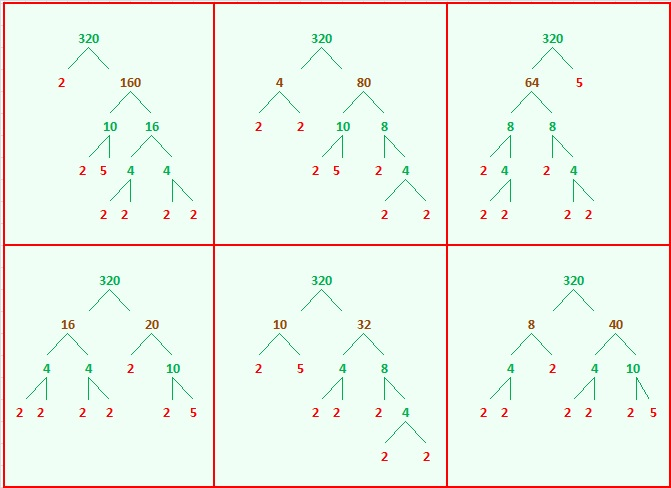Now try finding the factors in this Christmas tree puzzle that make it work like a multiplication table:Print the puzzles or type the factors on this excel file: 10 Factors 2014-12-08

A Logical Approach to FIND THE FACTORS: Find the column or row with two clues and find their common factor. Write the corresponding factors in the factor column (1st column) and factor row (top row).  Because this is a level three puzzle, you have now written a factor at the top of the factor column. Continue to work from the top of the factor column to the bottom, finding factors and filling in the factor column and the factor row one cell at a time as you go.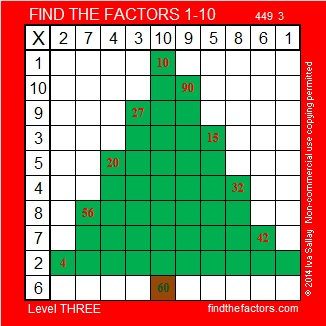# 318 Christmas Factor Tree Gif

• 318 is a composite number.
• Prime factorization: 318 = 2 x 3 x 53
• The exponents in the prime factorization are 1, 1, and 1. Adding one to each and multiplying we get (1 + 1)(1 + 1)(1 + 1) = 2 x 2 x 2 = 8. Therefore 318 has exactly 8 factors.
• Factors of 318: 1, 2, 3, 6, 53, 106, 159, 318
• Factor pairs: 318 = 1 x 318, 2 x 159, 3 x 106, or 6 x 53
• 318 has no square factors that allow its square root to be simplified. √318 ≈ 17.833This factoring tree gif solves and completes this Level ONE puzzle right before your eyes. Scroll down to see three possible factor trees for the number 318.make animated gifs like this at MakeAGif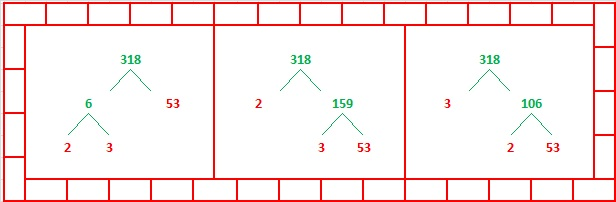Print the puzzles or type the factors on this excel file: 10 Factors 2014-12-08

# 316 Christmas Factor Trees

• 316 is a composite number.
• Prime factorization: 316 = 2 x 2 x 79, which can be written 316 = 2² x 79
• The exponents in the prime factorization are 2 and 1. Adding one to each and multiplying we get (2 + 1)(1 + 1) = 3 x 2  = 6. Therefore 316 has exactly 6 factors.
• Factors of 316: 1, 2, 4, 79, 158, 316
• Factor pairs: 316 = 1 x 316, 2 x 158, or 4 x 79
• Taking the factor pair with the largest square number factor, we get √316 = (√4)(√79) = 2√79 ≈ 17.776Today is all about Christmas trees. You can read how one family set up a beautiful Christmas tree at three-things-thursday-tree-edition, solve the Christmas tree puzzle, and scroll down to see two possible factor trees for 316.

Then polish up your shoes and boots. Place them near your front door or window so they will be ready to be filled with treats when Mikulás (St. Nickolas) makes his rounds tonight.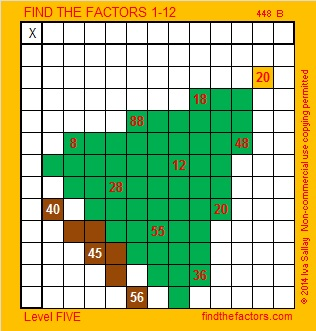Print the puzzles or type the factors on this excel file: 12 Factors 2014-12-01